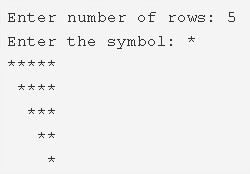# C Program Inverted Mirrored Right Triangle Star Pattern

C Program to print an inverted mirrored right triangle star pattern – In this article, we will discuss the multiple means to print an inverted mirrored right triangle star pattern.

Suitable examples and sample programs have also been added so that you can understand the whole thing very clearly. The compiler has also been added with which you can execute it yourself.

The means used in this particular piece are as follows:

• Using For Loop
• Using While Loop
• Using Do-While Loop

As it is known to everyone, a right triangle is a triangle which has at least one of its angles equal to 90 degrees.

The sum of the square of the adjacent sides of the right angle is equal to the square of the side that is opposite to the right angle.

An inverted mirrored right triangle looks like this:As you can see in the photo uploaded, you need to specify the number of rows and the symbol. Accordingly, the pattern will be printed after the code is executed.

Thus, the manifold ways used to print an inverted mirrored right triangle are as follows:

## Using For Loop

1. Store the number of rows value into the variable n.

2) Read the entered character using getchar() function, store that character into the character variable ch.

3) The outer for loop for(i=n;i>0;i–) iterates through rows.

4) The 1st inner for loop for(j=n-i;j>0;j–) iterates through columns and prints space.

5) The 2nd inner for loop for(j=0;j<i;j++)iterates through columns and prints symbol which is entered by user.

6) Cursor comes to next line after each iteration of outer for loop.

7) Each row contains n-i spaces.

Output:

### Using While Loop

1. i=n, The outer while loop iterates through rows until the condition i>0 becomes false.

2) j=n-i, The 1st inner while loop iterates through columns until the condition j>0 becomes false.

a) It prints space.

b) j value decreased by 1.

3) j=0, The 2nd inner while loop iterates through columns until the condition j<i becomes false.

a) It prints the symbol which is entered by the user.

b) j value increased by 1.

4) Cursor comes to next line.

5) i value decreased by 1. Again the outer loop checks the condition if it is true then the loop iterates again.

Output:

#### Using Do-While Loop

1. i=n, The outer do-while loop iterates through rows.

2) j=n-i

The outer do-while loop prints space using while loop with the condition j–>0.

Each row contains n-i spaces.

3) j=0, the inner do-while loop iterates through columns.

a) Prints symbol.

b) j value increased by 1.

c) Checks the condition j<i. If the condition is true then loop iterates again. Repeats until the condition fails.

4) Cursor comes to next line, i value decreased by 1.

5) Checks the condition of outer do-while loop i>0. If the condition is true the loop iterates again. Repeats until the condition fails.

Output:
x

## C Program To Check If Alphabet, Digit or Special Character | C Programs

C program to check for an alphabet, digit or special character – In this article, ...